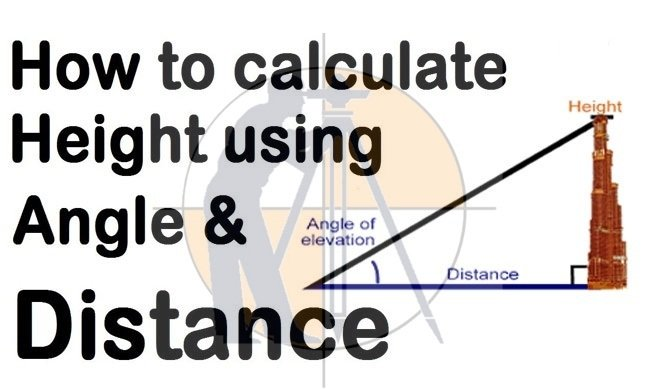Breaking News
Home / Land Surveying / Calculate Height In Surveying With Angle & Distance

# Calculate Height In Surveying With Angle & Distance

## How To Calculate Height In Surveying With Angle & Distance

In this Civil Engineering article, you will get some useful tips to determine the height of an object with angle and distance. The article is very useful for Surveyors.### The following formulas are used for calculation purpose:-

Sinθ = P/H (P stands for perpendicular and h stands for hypotenuse)

Cosθ = B/H (B stands for base)

Tanθ = P/B

### What is Scale Factor ?

It should be remembered that the opposite of the angle (in a triangle) is always perpendicular.

Here, the value of θ is taken as 45 degree and the value of base is taken as 10 meter

Now, Tanθ = P/B

### Now, after putting the values, we get the following result:-

tan450 = P/B = P/10

As we know the value of tan450 = 1

So, 1 = P/10 or P = 10

So, P = 10m

Now, Sinθ = P/H

### After putting the value, we get the following result:-

Sin450 = 10/H (as height is taken as 10m)

So, 0.707 = 10/H or H = 10/0.707 = 14.14m (value of Sin450 = 0.707)

Now, Cosθ = B/H

So, Cos450 = 10/H (as the value of base = 10)

So, 0.707 = 10/H or H = 10/0.707 = 14.14m

### THANKS.